Samacheer Kalvi 8th Maths Book Solutions Term 3 Chapter 3 – Geometry is available here. The Samacheer Kalvi 8th Maths book answers of Term 3 Chapter 3, available at BYJU’S, contain step by step explanations designed by our Mathematics experts. All these important questions are based on the new pattern prescribed by the Tamil Nadu board. Students can also get the solutions of other chapters on Samacheer Kalvi 8th Maths solutions. Practising these Samacheer Kalvi Class 8 Maths Book Term 3 Chapter 3 Questions and Solutions is the best way to prepare most proficiently for the final exams.

Term 3 Chapter 3 of the Samacheer Kalvi 8th Maths guide will help the students to solve problems related to the median of a triangle, centroid and its properties, altitude of a triangle, perpendicular bisector, circumcentre, angle bisector, incentre, construction of quadrilaterals.

### Samacheer Kalvi 8th Maths Term 3 Chapter 3: Geometry Book Exercise 3.1 Questions and Solutions

Question 1: In right triangle ABC, what is the length of altitude drawn from the vertex A to BC?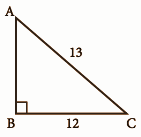Solution:

In this right-angled triangle ABC, the length of the altitude drawn from the vertex A is the leg AB itself.

By Pythagoras theorem,

AC2 = AB2 + BC2

132 = AB2 + 122

169 = AB2 + 144

169 – 144 = AB2

25 = AB2

AB = 5cm

Question 2: In triangle XYZ, YM is the angle bisector of ∠Y and ∠Y is 100°. Find ∠XYM and ∠ZYM.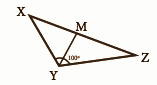Solution:

YM is the angle bisector of ∠Y

∠Y = 100°

The angle bisector divides the angle into two congruent angles.

∠XYM = ∠ZYM

∠Y = ∠XYM + ∠ZYM

100o = ∠XYM + ∠ZYM

Since ∠XYM = ∠ZYM,

2∠XYM = 100o

∠XYM = 50o

∠ZYM = 50o

Question 3: In triangle PQR, PS is a median and QS = 3.5 cm, then find QR?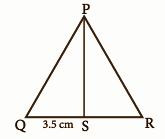Solution:

Given PS is the median and QS = 3.5cm

Median is the line drawn from a vertex to the midpoint of the opposite side.

QS = RS

QS = RS = 3.5cm

QR = QS + SR = 3.5 + 3.5 = 7cm

QR = 7cm

### Samacheer Kalvi 8th Maths Term 3 Chapter 3: Geometry Book Exercise 3.2 Questions and Solutions

Question 1: Identify the centroid of ∆PQR.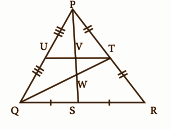Solution:

In triangle PQR, PT = TR

QT is the median from vertex Q.

QS = SR

PS is the median from vertex P.

QT and PS meet at W.

Hence, W is the centroid of the triangle PQR.

Question 2: Name the orthocentre of ∆PQR.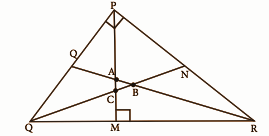Solution:

P = 90o

This is a right triangle.

Therefore, P is the orthocentre [In a right triangle, orthocentre is the vertex containing 90o.]

Question 3: In the given figure, A is the midpoint of YZ and G is the centroid of the triangle XYZ. If the length of GA is 3 cm, find XA.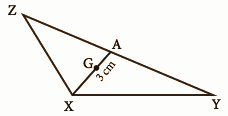Solution:

Given A is the midpoint of YZ.

ZA = AY

G is the centroid of △XYZ and it divides each median in a ratio 2:1.

XG : GA = 2:1

XG / GA = 2 / 1

XG / 3 = 2 / 1

XG = 2 * 3

XG = 6cm

XA = XG + GA

= 6 + 3

= 9cm

### Samacheer Kalvi 8th Maths Term 3 Chapter 3: Geometry Book Exercise 3.3 Questions and Solutions

Question 1: Construct the rhombus with the given measurements and find the area: FACE, FA = 6 cm and FC = 8 cm.

Solution: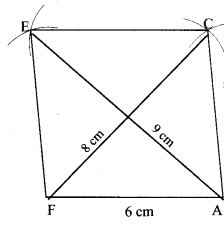Steps of construction:

1] A line segment FA = 6cm is drawn.

2] Two arcs of radii 8cm and 6cm are drawn with F and A as centres, which cuts at C.

3] Join FC and AC.

4] Two arcs of radii 6cm each is drawn with F and C as centres, which cuts at E.

5] Join FE and EC.

6] FACE is the required rhombus.

Area of rhombus = [1 / 2] * [d1] * [d2] = [1 / 2] * 8 * 9 = 36cm2

Question 2: Construct the rectangle with the given measurements and find the area: HAND, HA = 7 cm and AN = 4 cm.

Solution: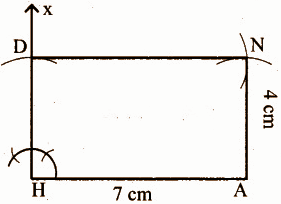Steps of construction:

1] A line segment HA = 7cm is drawn.

2] At H, construct HX perpendicular to HA.

3] An arc of radius 4cm is drawn with H as the centre which cuts at HX at D.

4] Two arcs of radii 4cm and 7cm are drawn with A and D as centres, which cuts at N.

5] Join AN and DN.

6] HAND is the required rectangle.

Area of rhombus = [l] * [b] = 7 * 4 = 28cm2

Question 3: Construct the square with the given measurement and find the area: EAST, EA = 6.5 cm.

Solution:Steps of construction:

1] A line segment EA = 6.5cm is drawn.

2] At E, construct EX perpendicular to EA.

3] An arc of radius 6.5cm is drawn with E as the centre which cuts at EX at T.

4] Two arcs of radii 6.5cm each is drawn with A and T as centres, which cuts at S.

5] Join TS and AS.

6] EAST is the required square.

Area of rhombus = a2 sq.units = (6.5)2 = 42.25 cm2